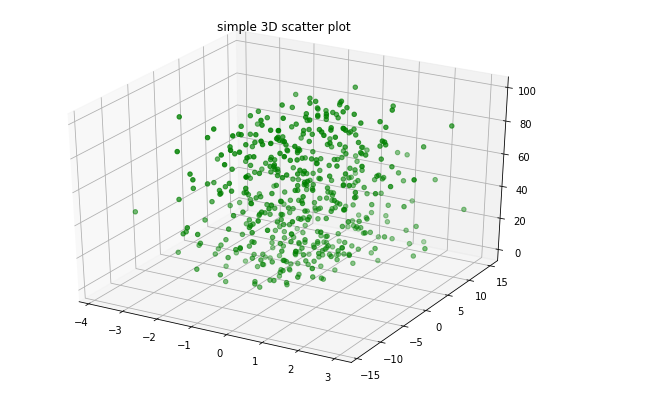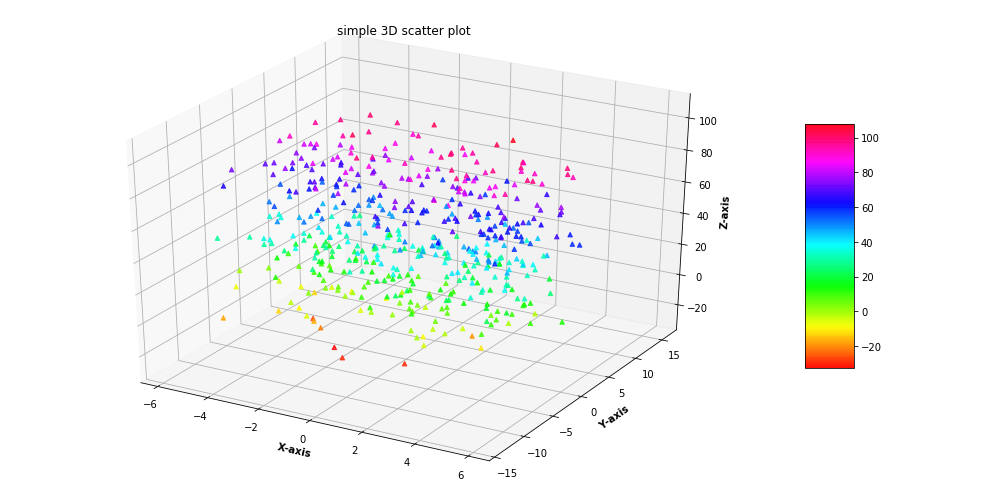# 3D Scatter Plotting in Python using Matplotlib

A 3D Scatter Plot is a mathematical diagram, the most basic version of three-dimensional plotting used to display the properties of data as three variables of a dataset using the cartesian coordinates.To create a 3D Scatter plot, Matplotlib’s mplot3d toolkit is used to enable three dimensional plotting.Generally 3D scatter plot is created by using ax.scatter3D() the function of the matplotlib library which accepts a data sets of X, Y and Z to create the plot while the rest of the attributes of the function are the same as that of two dimensional scatter plot.
Example 1: Let’s create a basic 3D scatter plot using the ax.scatter3D() function.

## Python3

 `# Import libraries` `from` `mpl_toolkits ``import` `mplot3d` `import` `numpy as np` `import` `matplotlib.pyplot as plt`     `# Creating dataset` `z ``=` `np.random.randint(``100``, size ``=``(``50``))` `x ``=` `np.random.randint(``80``, size ``=``(``50``))` `y ``=` `np.random.randint(``60``, size ``=``(``50``))`   `# Creating figure` `fig ``=` `plt.figure(figsize ``=` `(``10``, ``7``))` `ax ``=` `plt.axes(projection ``=``"3d"``)`   `# Creating plot` `ax.scatter3D(x, y, z, color ``=` `"green"``)` `plt.title(``"simple 3D scatter plot"``)`   `# show plot` `plt.show()`

Output :Example 2 : For better understanding Let’s take another example.

## Python3

 `# Import libraries` `from` `mpl_toolkits ``import` `mplot3d` `import` `numpy as np` `import` `matplotlib.pyplot as plt`     `# Creating dataset` `z ``=` `4` `*` `np.tan(np.random.randint(``10``, size ``=``(``500``))) ``+` `np.random.randint(``100``, size ``=``(``500``))` `x ``=` `4` `*` `np.cos(z) ``+` `np.random.normal(size ``=` `500``)` `y ``=` `4` `*` `np.sin(z) ``+` `4` `*` `np.random.normal(size ``=` `500``)`   `# Creating figure` `fig ``=` `plt.figure(figsize ``=` `(``16``, ``9``))` `ax ``=` `plt.axes(projection ``=``"3d"``)` `  `  `# Add x, y gridlines ` `ax.grid(b ``=` `True``, color ``=``'grey'``, ` `        ``linestyle ``=``'-.'``, linewidth ``=` `0.3``, ` `        ``alpha ``=` `0.2``) `     `# Creating color map` `my_cmap ``=` `plt.get_cmap(``'hsv'``)`   `# Creating plot` `sctt ``=` `ax.scatter3D(x, y, z,` `                    ``alpha ``=` `0.8``,` `                    ``c ``=` `(x ``+` `y ``+` `z), ` `                    ``cmap ``=` `my_cmap, ` `                    ``marker ``=``'^'``)`   `plt.title(``"simple 3D scatter plot"``)` `ax.set_xlabel(``'X-axis'``, fontweight ``=``'bold'``) ` `ax.set_ylabel(``'Y-axis'``, fontweight ``=``'bold'``) ` `ax.set_zlabel(``'Z-axis'``, fontweight ``=``'bold'``)` `fig.colorbar(sctt, ax ``=` `ax, shrink ``=` `0.5``, aspect ``=` `5``)`   `# show plot` `plt.show()`

Output :My Personal Notes arrow_drop_upCheck out this Author's contributed articles.

If you like GeeksforGeeks and would like to contribute, you can also write an article using contribute.geeksforgeeks.org or mail your article to contribute@geeksforgeeks.org. See your article appearing on the GeeksforGeeks main page and help other Geeks.

Please Improve this article if you find anything incorrect by clicking on the "Improve Article" button below.